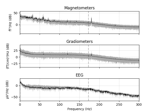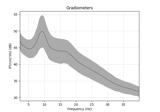# mne.time_frequency.psd_multitaper¶

mne.time_frequency.psd_multitaper(inst, fmin=0, fmax=inf, tmin=None, tmax=None, bandwidth=None, adaptive=False, low_bias=True, normalization='length', picks=None, proj=False, n_jobs=1, verbose=None)[source]

Compute the power spectral density (PSD) using multitapers.

Calculates spectral density for orthogonal tapers, then averages them together for each channel/epoch. See  for a description of the tapers and  for the general method.

Parameters
instinstance of Epochs or Raw or Evoked

The data for PSD calculation.

fminfloat

Min frequency of interest.

fmaxfloat

Max frequency of interest.

tmin

Min time of interest.

tmax

Max time of interest.

bandwidthfloat

The bandwidth of the multi taper windowing function in Hz. The default value is a window half-bandwidth of 4.

Use adaptive weights to combine the tapered spectra into PSD (slow, use n_jobs >> 1 to speed up computation).

low_biasbool

Only use tapers with more than 90% spectral concentration within bandwidth.

normalizationstr

Either “full” or “length” (default). If “full”, the PSD will be normalized by the sampling rate as well as the length of the signal (as in nitime).

picks

Channels to include. Slices and lists of integers will be interpreted as channel indices. In lists, channel type strings (e.g., ['meg', 'eeg']) will pick channels of those types, channel name strings (e.g., ['MEG0111', 'MEG2623'] will pick the given channels. Can also be the string values “all” to pick all channels, or “data” to pick data channels. None (default) will pick good data channels(excluding reference MEG channels).

projbool

Apply SSP projection vectors. If inst is ndarray this is not used.

n_jobsint

The number of jobs to run in parallel (default 1). Requires the joblib package.

verbose

If not None, override default verbose level (see mne.verbose() and Logging documentation for more). If used, it should be passed as a keyword-argument only.

Returns
psdsndarray, shape (…, n_freqs)

The power spectral densities. If input is of type Raw, then psds will be shape (n_channels, n_freqs), if input is type Epochs then psds will be shape (n_epochs, n_channels, n_freqs).

freqsndarray, shape (n_freqs,)

The frequencies.

Notes

New in version 0.12.0.

References

1

Slepian, D. “Prolate spheroidal wave functions, Fourier analysis, and uncertainty V: The discrete case.” Bell System Technical Journal, vol. 57, 1978.

2

Percival D.B. and Walden A.T. “Spectral Analysis for Physical Applications: Multitaper and Conventional Univariate Techniques.” Cambridge University Press, 1993.

## Examples using mne.time_frequency.psd_multitaper¶